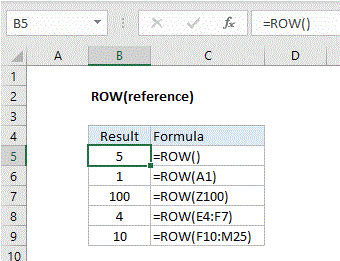## Excel Office

Excel How Tos, Tutorials, Tips & Tricks, Shortcuts

# How to use Excel ROW Function

This Excel tutorial explains how to use the ROW function with syntax and examples.

## Excel ROW Function Description

Microsoft Excel ROW function returns the row number of a cell reference.

The ROW function is a built-in function in Excel that is categorized as a Lookup/Reference Function. The ROW function can be entered as part of a formula in a cell of a worksheet.

Worked Example:   How to get relative row numbers in a range in ExcelExplanation: The Excel ROW function returns the row number for a reference. For example, ROW(C5) returns 5, since C5 is the fifth row in the spreadsheet. When no reference is provided, ROW returns the row number of the cell which contains the formula.

Worked Example:   How to strip non-numeric characters in Excel

ROW function is used to get the row number for a cell reference or a range. For example:

```=ROW(A3) // returns 3
=ROW(D5) // returns 5
=ROW(F10:J15) // returns 10```

## Returns

The ROW function returns a numeric value.

Note: If the reference parameter is omitted, it assumes that the reference is the cell address in which the ROW function has been entered in.